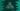# Dart program to check if an integer is odd or even# Introduction :

Dart integer class contains two properties to check if a number is even or odd. We can check these properties to find out quickly if a number is odd or even. In this tutorial, I will show you how to do that :

## isEven and isOdd :

isEven and isOdd are two boolean values that you can access in an integer variable in dart. Just check for these values to find out if a number is odd or even.

In the below example, we will take one number as input from the user and find out if it is odd or even.

## Example program :

``````import 'dart:io';

void main() {
int number;

print("Enter a number : ");

if (number.isEven) {
print("\$number is an even number");
} else if (number.isOdd) {
print("\$number is an odd number");
}
}``````

### Sample Output :

``````Enter a number :
12
12 is an even number

Enter a number :
13
13 is an odd number``````

Note that it will also work for negative numbers. For example, if your number is -12, it will print as an even number.